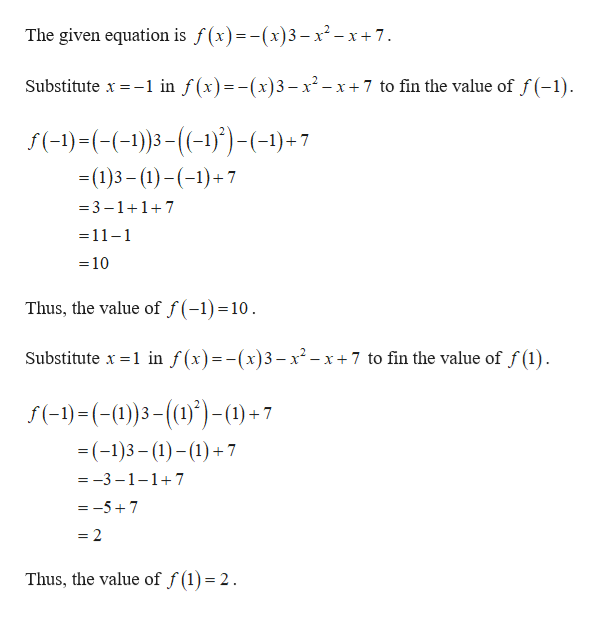F(x)=(-x)3-x2-x+7 we are supposed to indicate the function value with f(1)+f(-1). I know the answer is 12 but am having issues reaching that in my work.

Question

F(x)=(-x)3-x2-x+7 we are supposed to indicate the function value with f(1)+f(-1). I know the answer is 12 but am having issues reaching that in my work.

Step 1help_outlineImage TranscriptioncloseThe given equation is f(x)=-(x)3- x2-x+7. in f(x)=-(x)3-x2-x7 to fin the value of f(-1) Substitute x f(-)-((-1)3(-))-(-1)+7 =(1)3 (1))7 3-117 =11-1 =10 Thus, the value of f(-1) =10 Substitute x 1 in f(x)=-(x)3- x2- x+7 to fin the value of f(1) f(-1)(-0))3())-1)+7 =(-1)3(1)17 =-3-1-1+7 =-57 = 2 Thus, the value of f (1) = 2 fullscreen

Want to see the full answer?

See Solution

Want to see this answer and more?

Our solutions are written by experts, many with advanced degrees, and available 24/7

See Solution
Tagged in

Polynomials